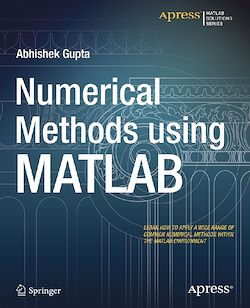# Numerical Methods using MATLAB

-English
137 Pages

Description

Numerical Methods with MATLAB provides a highly-practical reference work to assist anyone working with numerical methods. A wide range of techniques are introduced, their merits discussed and fully working MATLAB code samples supplied to demonstrate how they can be coded and applied.

Numerical methods have wide applicability across many scientific, mathematical, and engineering disciplines and are most often employed in situations where working out an exact answer to the problem by another method is impractical.

Numerical Methods with MATLAB presents each topic in a concise and readable format to help you learn fast and effectively. It is not intended to be a reference work to the conceptual theory that underpins the numerical methods themselves. A wide range of reference works are readily available to supply this information. If, however, you want assistance in applying numerical methods then this is the book for you.

Subjects

##### IT systems

Informations